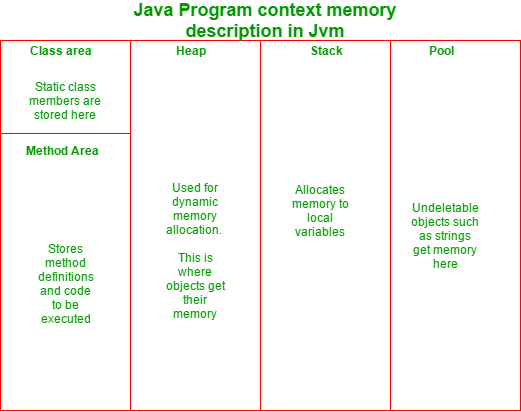# How to prevent objects of a class from Garbage Collection in Java

• Difficulty Level : Easy
• Last Updated : 29 Nov, 2018

The garbage collector in Java is automatic, i.e the user doesn’t have to manually free an occupied memory which was dynamically allocated. And how does a garbage collector decide which object is to be deleted? It’s simple: the object which loses it’s reference, is marked for deletion from the heap memory. For example, look at the following piece of code:

 `// Java code to demonstrate when an object would ` `// be deleted by the garbage collector ` ` `  `class` `A { ` `    ``void` `f() ` `    ``{ ` `        ``A x = ``new` `A(); ``/*object created locally ` `              ``It's scope remains valid till the ` `              ``termination of this function*/` `    ``} ` `    ``public` `static` `void` `main(String a[]) ` `    ``{ ` `        ``f(); ` ` `  `        ``/* function f() terminates,  ` `           ``and hence the object 'x'  ` `           ``too gets collected  ` `           ``by the garbage collector*/` `    ``} ` `} `

The above code shows that the reference variable ‘x’ had a scope limited to the function ‘f()’. Therefore, after the termination of the mentioned function, the variable too has no recognition. Therefore, the object created loses it’s reference, and hence, is collected by the garbage collector.

The objects that are collected by the garbage collector, are collected so as to free the heap memory, where dynamic memory is allocated. The following diagram explains the different memory segments in a Java program, and their uses:However, sometimes the question often arises: ‘Is there a way by which we can prevent an object from being collected by the garbage collector?’

There are several ways which can make objects undeletable in Java. They are discussed below:

By Increasing Heap Memory

Java allocates memory to it’s object in a partition called ‘heap’ (except for String and some other special objects which are allocated memory in pool). Since the heap memory is limited, some memory is always needed to be freed to accommodate space for new objects. However, we can, to some limit make objects undeletable by increasing the heap size. The following jvm command line parameters can get the job done:

• Xms
• xmx
• Xmx specifies the maximum memory allocation pool for a Java virtual machine (JVM), while Xms specifies the initial memory allocation pool. The following is an example:

`java -Xms256m -Xmx2048m classfile`

Here, in the above example, you start with 256 MB of initially allocated heap memory, which could be expanded to a maximum of 2048MB of heap size.

This approach leads to the garbage collector running infrequently, however when it runs, will take longer than before to complete the garbage collection task.

By using Singleton Class

In case of a singleton class, the reference of the only object created can be stored in a static reference. Since static members are stored in class area (a memory segment), their lifetime spans the lifetime of the program. The following program explains how to do it:

 `public` `class` `Singleton { ` ` `  `    ``/* static class member which  ` `       ``will store the object reference*/` `    ``private` `static` `Singleton uniqueInstance; ` ` `  `    ``private` `Singleton() ` `    ``{ ` `    ``} ` ` `  `    ``public` `static` `synchronized` `Singleton getInstance() ` `    ``{ ` `        ``if` `(uniqueInstance == ``null``) { ` `            ``uniqueInstance = ``new` `Singleton(); ` `        ``} ` `        ``return` `uniqInstance; ` `    ``} ` `} `

The above example shows that since the object’s reference has been passed into a static variable, it will never be lost. Therefore, the object won’t be deleted until the end of the program. However, the only issue remains that only one object can be created here.

Using public void finalise()

Finalize is a call-back method (a method called by the JVM, and not by the user), that is last method executed on an object. A subclass may override the finalize method to dispose of system resources or to perform other cleanup.

How to prevent garbage collection using finalize?

The finalize method of a class can be overridden to preserve the reference of the object that is about to be deleted. The following program demonstrates, how:

 `// Java code to demonstrate how to prevent garbage collection ` `// of an object using finalize method ` `class` `A { ` `    ``static` `A y; ` `    ``void` `f() ` `    ``{ ` `        ``A x = ``new` `A(); ` `    ``} ` `    ``pubic ``void` `finalize() ` `    ``{ ` `        ``y = ``this``; ``// Putting the reference id ` `        ``// of the current object ` `        ``// into the static variable y ` ` `  `        ``System.out.println("Object reference saved. The object ` `              ``won't be collected by the garbage collector"); ` `    ``} ` `    ``public` `static` `void` `main(String a[]) ` `    ``{ ` `        ``f(); ``// function called ` `    ``} `

Output:

`Object reference saved. The object won't be collected by the garbage collector`

As explained previously, finalize is the last method that is executed on an object. In the above program, the function ‘f()’ creates a local object of class A. When the call of the function terminates, the scope of the variable ‘x’ terminates as well, and hence, is marked for collection by the garbage collector. However, before the garbage collector could delete the object, finalize runs.

Inside the body of the method ‘finalize’, one can see that the static member of class A, ‘y’, is assigned the reference id of the current object. As a result, the reference id of the object to be deleted is preserved, and hence, the object is not deleted.

However, it is really important to know that making an object undeletable is risky, and increases the chances of memory leaks. Therefore, it is not advisable to do so.

My Personal Notes arrow_drop_up
Recommended Articles
Page :